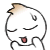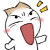VU HELP

Virtual University of Pakistan Study forum !

assignment num.2

Q 1. The 3rd and 4th term of geometric progressions are 12 and 8 respectively find sum of infinity of the progression?

Q  2. in how many ways can a team of 6 candidates can be formed out of a total 10 candidates such that 2 particular candidates should  be included in each  department?

plz give the solution plzzzzzzzzzViews: 742

Replies to This Discussion

Ialso needy

que 1solution

3rd term=12
4th term=8
Nth term=ar^n-1
12=ar^2..i
8=ar^3..ii
divide through to eliminate a
3/2=r^-1
Solving i have
r=2/3
⇒12=ar^2..from i
12=a*4/3
a=27

a=27
r=2/3
S∞=a/(1-r)
=81

students u can try it by your own....

And the second q
this may be helpful for u......am not sure that that,s correct solution..
que 2:
We have 6 slots to fill. Let's call them Slot A, Slot B, Slot C, Slot D, Slot E, Slot F.

The first two slots (A and B) are reserved for these two particular candidates who must be on the team.
So we go from 6 slots to 4 slots (6-2=4).

The pool of 10 candidates reduces to 8 candidates (10-2 = 8).

So we have 4 slots (C,D,E,F) and a pool of 8 people to choose from.

Slot C has 8 choices.
Slot D has 7 choices.
Slot E has 6 choices.
Slot F has 5 choices.

There are 8*7*6*5 = 1680 ways to fill those four slots (given a pool of 8 people to choose from).
This result would be the answer if order mattered, but it doesn't.

In this case order does NOT matter because a team like {x,y,z,w} is the same as {w,z,y,x}. The letters represent the team members.
All we care about is the overall team and not the order of any members on any given team. Each team member is equal.
Turns out that there are 4! = 4*3*2*1 = 24 different ways to arrange any one team of 4 people.
Since order doesn't matter, we divide the previous result by 24 to get 1680/24 = 70
This means that there are 70 different teams of 4 people (drawn from a pool of 8).

Side Note: we can use the combination formula to get the same result
C(n,r) = (n!)/(r!(n-r)!)
C(8,4) = (8!)/(4!*(8-4)!)
C(8,4) = (8!)/(4!*4!)
C(8,4) = (8*7*6*5*4!)/(4!*4!)
C(8,4) = (8*7*6*5)/(4!)
C(8,4) = (8*7*6*5)/(4*3*2*1)
C(8,4) = (1680)/(24)
C(8,4) = 70

Now let's incorporate the two reserved individuals in slots A and B.
We have 2 people filling 2 slots giving 1 way to do this.
Again the order doesn't matter.

So there are 1*70 = 70 different ways to fill all 6 slots according to the conditions provided.